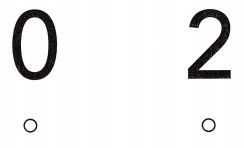# Texas Go Math Kindergarten Module 2 Assessment Answer Key

Refer to our Texas Go Math Kindergarten Answer Key Pdf to score good marks in the exams. Test yourself by practicing the problems from Texas Go Math Kindergarten Module 2 Assessment Answer Key.

## Texas Go Math Kindergarten Module 2 Assessment Answer Key

Concepts and Skills

DIRECTIONS: 1-2. Write the number of counters in the five frame. (TEKS K.2.B) 3-4. Write the number of counters that are shown. (TEKS K.2.D )

Question 1.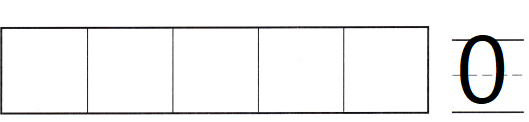Explanation:
Written the number of counters in the five frame.
There are 0 counters

Question 2.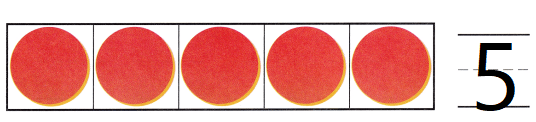Explanation:
Written the number of counters in the five frame.
There are 5 counters

Question 3.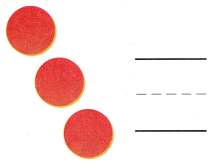Explanation:
There are 3 circles
traced and written the number 3

Question 4.Explanation:
There are 5 circles
traced and written the number 5

DIRECTIONS: 5. Write how many cubes are in each tower. Write the numbers in order. (TEKS K.2.A) 6. Choose the correct answer. There are two apples on the table. Kia takes the two apples to school. How many apples are on the table now? (TEKS K.2.B)

Question 5.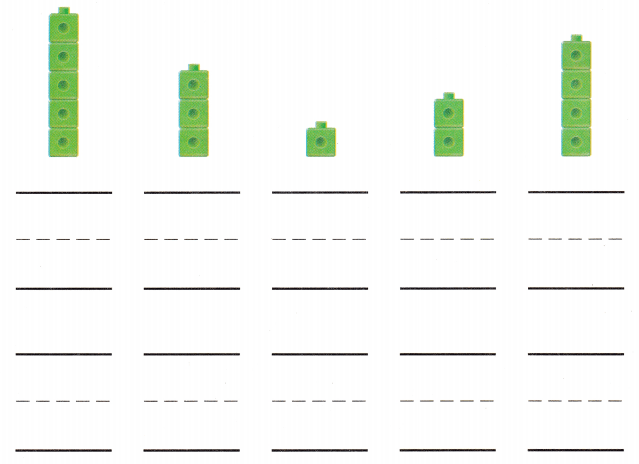Explanation:
Written the number of cubes
an written them in order

Question 6.
Texas Test Prep